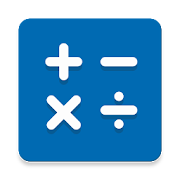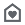# NT Calculator - Extensive Calculator ProEveryone
2,307
Dear you,

This Extensive Calculator app allows you to easily handle all the calculations necessary for everyday life with a single application. A Calculator app with a simple interface and practical functions! It can replace your default calculator.

This calculator app don't require ANY PERMISSION on your phone. And it is light and fast.

With Extensive Calculator Pro on your Android, you do not need to bring your calculator anymore. This calculator app serves you everything from A - Z.

List of calculators currently supported:
1. Standard Calculator
• Quick and easy.
• Basic features such as: %, +, -, x, /, 0 - 9, ( and ).
• Memory function such as: M+, M-, MR, MC.

2. Scientific Calculator
• All features such as: sin, cos, tan, %, !, 0-9, (, ), RAD and DEG, log, ln, Mod, +, -, x, /, SQRT, SQR, signed,...
• You can use EXP is E (sam as 10 ^ ) to calculate large decimal number. If you need to get previous result of calculator, you can use ANS button.

• Covert from one thing to the other things is very simple.
• Base 2, 8 ,10, 16

4. Time Calculator
• Three primary function is hour (h), minute (m), second (s), millisecond (s) with basic features such as: %, +, -, x, /, 0 - 9, ( and ).
• History available. And you can reuse by pressing calculation history (this feature is available in standard, scientific and time calculator).

5. Loan Calculator
• Suitable for car, bank loan
• Three primary input are loan amount, interest rate, loan term.

6. Date Calculator
• Calculate difference between of two date.

7. Unit Converter
Supports many Unit of measurement commonly used in everyday life such as:
• Weight converter: kg to st, lbs to grams, lbs to kilograms, ...
• Length converter: size converter, meters to kilometers, ...
• Speed converter: km/h, cm/s, m/s, m/s to km/h, ...
• Temperature converter: c to f, f to c, c to kelvin, ...
• Data converter: byte to bit, bit to byte, ...
• Area converter: km2 to m2, 1 hectare to km2, cm2 to m2, ...
• Time converter: minute to second, year to second, ...
• Energy converter: joule, kwh, ...
• Pressure converter: atmospheres, bars, kilopascals, pascals, ...
• Volume converter: gallons to qts, teaspoon, cups, liters, cubic feet, ...
• Fuel Converter: many units,...

8. Support function in this calculator
• Format number type: 1,234.56 or 1.234,56.
• Percentage method calculation:
- 100 + 5% = 105 and 100 x 5% = 500.
- 100 + 5% = 100.5 and 100 x 5% = 5.
- 100 + 5% = 100.05 and 100 x 5% = 5.
• Rounding number: from 0 to 10.
• Operation priority:
- 2 + 2 x 2 = 6 (OP).
- 2 + 2 x 2 = 8 (WOP).

Thank you for using the Calculator app, if you have questions I am always ready to answer you. I think you will find this app useful and use it with pleasure.

The list will be increased!

Collapse

## Reviews

Review policy and info
4.3
2,307 total
5
4
3
2
1

## What's New

Thank you for choosing this app <3 What's new:
1. Memory functions: M+, M-, MC, MR for standard calculator.
2. Add percentage method calculation: "100+5%=105; 100×5%=500", "100+5%=105; 100×5%=5" or "100+5%=100.05; 100×5%=5" (You can change in app's settings).
3. Add Danish, Swedish, Finnish, Ukraine language.
4. Fix cannot change language in app's settings.
Collapse

Eligible for Family LibraryEligible if bought after 7/2/2016. Learn More
Updated
September 4, 2020
Size
2.8M
Installs
100,000+
Current Version
3.6.2
Requires Android
5.0 and up
Content Rating
Everyone
Permissions
Offered By
Lê Trần Ngọc Thành (kineita)
Developer
Postal: 700000 No.32 Street 9, Ward 4, District 4, Ho Chi Minh City, VietnamSee more